Download Presentation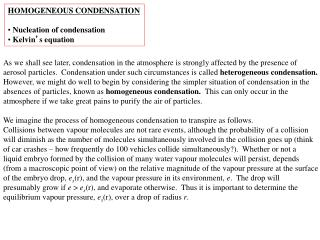HOMOGENEOUS CONDENSATION Nucleation of condensation Kelvin ’ s equation

# HOMOGENEOUS CONDENSATION Nucleation of condensation Kelvin ’ s equation - PowerPoint PPT Presentation

HOMOGENEOUS CONDENSATION Nucleation of condensation Kelvin ’ s equation. As we shall see later, condensation in the atmosphere is strongly affected by the presence of aerosol particles. Condensation under such circumstances is called heterogeneous condensation.I am the owner, or an agent authorized to act on behalf of the owner, of the copyrighted work described.
Download Presentation## HOMOGENEOUS CONDENSATION Nucleation of condensation Kelvin ’ s equation

An Image/Link below is provided (as is) to download presentation

Download Policy: Content on the Website is provided to you AS IS for your information and personal use and may not be sold / licensed / shared on other websites without getting consent from its author.While downloading, if for some reason you are not able to download a presentation, the publisher may have deleted the file from their server.

- - - - - - - - - - - - - - - - - - - - - - - - - - E N D - - - - - - - - - - - - - - - - - - - - - - - - - -
Presentation Transcript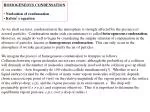HOMOGENEOUS CONDENSATION

• Nucleation of condensation
• Kelvin’s equation

As we shall see later, condensation in the atmosphere is strongly affected by the presence of

aerosol particles. Condensation under such circumstances is called heterogeneous condensation.

However, we might do well to begin by considering the simpler situation of condensation in the

absences of particles, known as homogeneous condensation. This can only occur in the

atmosphere if we take great pains to purify the air of particles.

We imagine the process of homogeneous condensation to transpire as follows.

Collisions between vapour molecules are not rare events, although the probability of a collision

will diminish as the number of molecules simultaneously involved in the collision goes up (think

of car crashes – how frequently do 100 vehicles collide simultaneously?). Whether or not a

liquid embryo formed by the collision of many water vapour molecules will persist, depends

(from a macroscopic point of view) on the relative magnitude of the vapour pressure at the surface

of the embryo drop, es(r), and the vapour pressure in its environment, e. The drop will

presumably grow if e > es(r), and evaporate otherwise. Thus it is important to determine the

equilibrium vapour pressure, es(r), over a drop of radius r.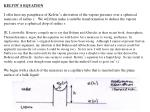KELVIN’S EQUATION

I offer here my paraphrase of Kelvin’s derivation of the vapour pressure over a spherical meniscus of radius r. We will then make a suitable transformation to deduce the vapour pressure over a spherical drop of radius r.

[E. Lozowski: Honesty compels me to say that Bohren and Albrecht, in their recent book, Atmospheric Thermodynamics, argue that the equation we will derive for a meniscus is wrong, and hence, by implication, that Kelvin’s original derivation may have been wrong. Although I cannot at present find an error in their argument, my intuition is that Bohren and Albrecht may have derived a correct result but applied it incorrectly (of course I could be wrong too). So for now, I will leave my derivation as is. Suffice to say that the end result for the vapour pressure over a droplet is the same as that derived by Bohren and Albrecht. And no-one seems to contest Kelvin’s equation for a liquid drop. So my result is widely accepted, even though some might argue that the method I used to get it isn’t.]

We begin with a sketch of the meniscus in a capillary tube that is inserted into the plane surface of a bulk liquid: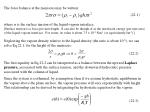The force balance at the meniscus may be written:

where  is the surface tension of the liquid-vapour interface.

[Surface tension is a force per unit length. It can also be though of as the interfacial energy (per unit area) of the liquid-vapour interface. For water, its value is about 7.5 x 10-2 Nm-1 (or equivalently Jm-2).]

Neglecting the vapour density relative to the liquid density (the ratio is about 10-5), we can solve Eq 22.1, for the height of the meniscus:

(22.1)

(22.2)

The first equality in Eq 22.2 can be interpreted as a balance between the upward Laplace pressure, associated with the surface tension, and the downward hydrostatic pressure associated with the column of liquid.

Since the system is isothermal, by assumption, then if we assume hydrostatic equilibrium in the vapour above the plane surface, the vapour pressure will vary exponentially with height. This relationship can be derived by integrating the hydrostatic equation for the vapour.

(22.3)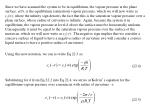Since we have assumed the system to be in equilibrium, the vapour pressure at the plane surface, e(0), is the equilibrium (saturation) vapour pressure, which we will now write as es(), where the infinity sign denotes the fact that this is the saturation vapour pressure over a plane surface, whose radius of curvature is infinite. Again, because the system is in equilibrium, the vapour pressure at level h above the surface must be horizontally uniform. Consequently, it must be equal to the saturation vapour pressure over the surface of the meniscus, which we will now write as es(-r). The negative sign implies that we consider a concave surface of liquid to have a negative radius of curvature (we will consider a convex liquid surface to have a positive radius of curvature).

Using this new notation, we can re-write Eq 22.3 as:

Substituting for h from Eq 22.2 into Eq 22.4, we arrive at Kelvin’s equation for the equilibrium vapour pressure over a meniscus with radius of curvature –r:

(22.4)

(22.5)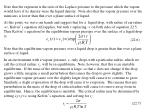Note that the exponent is the ratio of the Laplace pressure to the pressure which the vapour would have if its density were the liquid density. Note also that the vapour pressure over the meniscus is lower than that over a plane surface of liquid.

At this point, we wave our hands and suggest that for a liquid drop, with radius of curvature +r, Kelvin’s equation still applies, but with r replacing –r on both sides of equation 22.5. Thus Kelvin’s equation for the equilibrium vapour pressure over the surface of a liquid drop is:

Note that the equilibrium vapour pressure over a liquid drop is greater than that over a plane surface of liquid.

In an environment with a vapour pressure, e, only drops with a particular radius, which we call the critical radius, rc, will be in equilibrium. Note, however, that this is an unstable equilibrium. If the mass of the environment is large, so that e does not change if the drop grows a little, imagine a small perturbation that causes the drop to grow slightly. The equilibrium vapour pressure over the slightly larger drop will cause it to continue to grow. Similarly, a slight evaporation of the drop will cause it to evaporate. This means that any perturbation in the mass of the drop of critical radius will cause it to move away from its equilibrium. Hence, the equilibrium is unstable. The critical radius may be determined by setting es(rc)=e, using Kelvin’s equation, and solving for rc:

(22.6)

(22.7)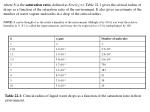where S is the saturation ratio, defined as S=e/es(). Table 22.1 gives the critical radius of

drops as a function of the saturation ratio of the environment. It also gives an estimate of the

number of water vapour molecules in a drop of the critical radius.

NOTE: S can be thought of as the relative humidity of the environment. Multiply it by 100 if you want the relative

humidity in %. S-1 is called the supersaturation, and it may also be expressed as a % by multiplying it by 100.

Table 22.1: Critical radius of liquid water drops as a function of the saturation ratio in their

environment.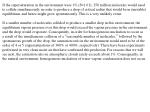If the supersaturation in the environment were 1% (S=1.01), 250 million molecules would need

to collide simultaneously in order to produce a drop of critical radius that would be in (unstable)

equilibrium, and hence might grow spontaneously. This is a very unlikely event.

If a smaller number of molecules collided to produce a smaller drop in this environment, the

equilibrium vapour pressure over this drop would exceed the vapour pressure in the environment,

and the drop would evaporate. Consequently, in order for homogeneous nucleation to occur as

a result of the simultaneous collision of a “reasonable number of molecules,” followed by the

spontaneous growth of the drop, the saturation ratio in the environment would need to be of the

order of 4 or 5 (supersaturations of 300% or 400%, respectively). There have been experiments

performed in very clean moist air that have confirmed this prediction. For reasons that we will

see next, the saturation ratio in atmospheric clouds rarely exceeds about 1%. Consequently, in

the natural environment, homogeneous nucleation of water vapour condensation does not occur.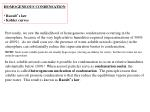HOMOGENEOUS CONDENSATION

• Raoult’s law
• Köhler curves

Previously, we saw the unlikelihood of homogeneous condensation occurring in the atmosphere, because of the very high relative humidities required (supersaturations of 300% or 400%). As we shall soon see, the presence of water-soluble aerosols (particles) in the atmosphere can substantially reduce this supersaturation barrier to condensation.

NOTE: Such water-soluble particles are usually hygroscopic ( having an affinity for water), but the two terms are not entirely equivalent.

In fact, soluble aerosols can make it possible for condensation to occur at relative humidities substantially below 100%! When aerosol particles serve as condensation nuclei, the process is called heterogeneous nucleation of condensation. The principle reason that soluble aerosols promote condensation is that they reduce the equilibrium vapour pressure of pure water. This result is known as Raoult’s law.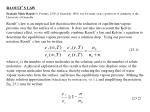RAOULT’S LAW

François Marie Raoult (b. Fournes, 1830, d. Grenoble, 1901) was for many years a professor of chemistry at the University of Grenoble.

Raoult’s law is an empirical law that describes the reduction of equilibrium vapour pressure over the flat surface of a solution. It does not take into account the Kelvin (curvature) effect, so we will subsequently combine Raoult’s law and Kelvin’s equation to determine the equilibrium vapour pressure over a solution drop. Using our previous notation, Raoult’s law can be written:

where no is the number of water molecules in the solution, and n is the number of solute molecules.A physical explanation of this result is that solute ions displace some of the water vapour molecules from the surface, thereby reducing the outgoing flux of water vapour molecules from the surface, and hence the equilibrium vapour pressure. Making the dilute solution approximation [which may be written n/no << 1.], and simplifying the notation, Eq. 23.1 may be written:

(23.1)

(23.2)We may determine n, no as follows:

where NA is Avogadro’s number, Mv and Ms are, respectively, the molecular weights of water and the solute, and ms are, respectively, the masses of liquid water and the solute, and i is known as the van’t Hoff factor, which is a small integer “fudge factor”, intended to take into account the degree of ionic dissociation of the solute.

NOTE: For NaCl, the van’t Hoff factor is 2, because n moles of Sodium Chloride dissolve into n moles of Sodium ions and another n moles of Chloride ions, for a total of 2n moles.

Substituting Eq. 23.3 into Eq. 23.2 and simplifying:

(23.3)

(23.4)KÖHLER CURVES

Kelvin’s equation may be written as:

[The approximation in Eq 23.5 applies when a/r <<1. As an exercise, you should determine for yourself what is the minimum drop size for which this approximation can be used.]

Multiplying Eqs 23.4 and 23.5, leads to:

where the last inequality defines the saturation ratio, . [Not to be confused with the lapse rate!] The plot of (r), for a particular solute mass, is known as a Köhler curve, as in the following diagram.

The peak in the Köhler curve is the point (r*, S*) where and .

It is at this point that the equilibrium changes from a stable to an unstable one.

(23.5)

(23.6)ATMOSPHERIC AEROSOLS

• Junge distribution
• Cloud condensation nuclei
• Activity spectrum

Previously, we saw the potential importance of atmospheric aerosols for condensation in the atmosphere. Not all atmospheric aerosols, however, act as cloud condensation nuclei. So we will begin with some overall characteristics of atmospheric aerosols, and then focus in on those that are considered to be responsible for promoting condensation in clouds.

JUNGE DISTRIBUTION

Let us begin with their size distribution. Atmospheric aerosols range in size from about 10-3m to about 10 m . The smallest size corresponds to particles that have been produced by “gas-to-particle conversion” processes. [These include condensation of hot vapours and chemical conversion of gases to low volatility vapours.]

The largest size is determined by the settling velocity (terminal velocity) of aerosol particles. Some representative values are given in the table below.Table 24.1: Settling velocity of atmospheric aerosols of density 1000 kg/m3.

Atmospheric aerosol particles with diameters much greater than 10 m are likely to settle out

in about a day or less. Hence they are rarely found in the atmosphere, except locally for short

periods of time (e.g., in the gusty winds of thunderstorm outflows).

In order to understand aerosol measurements, we will need to begin with a few fundamentals

about how size distributions are represented mathematically. We will let n(r)dr be the number

of aerosol particles per unit volume in the size range r to r+dr. The function n(r) is called the

number density function. Then the number of aerosol particles per unit volume, of size r

is given by the number distribution function, N(r), where:(24.1)

Because atmospheric aerosol sizes range over four orders of magnitude, it is convenient to plot

the size distributions as a function of the logarithm of particle radius. Hence we can define the

logarithmic number density functionnl(r) as follows:

(24.2)

where now nl(r)dlnr is the number of aerosol particles per unit volume with radii from r to r+dr.

NOTE: Just to confuse matters, some scientists use logarithms to base 10 rather than natural logarithms. Others define

N(r) as the number of particles per unit volume with radii  r, in which case the negative sign on the right hand side of

Eq. 24.2 disappears.

As the attached graph shows (Fig. 4.1 from F.H. Ludlam’s book Clouds and Storms), the

logarithmic density function of atmospheric aerosols depends, not surprisingly, on where the

measurements are made. The total concentration (number per unit volume) of aerosols, which is

the total area under the logarithmic density function curve, increases as one moves towards the

surface (from the stratosphere into the troposphere), and as one moves from maritime to

continental air masses. The table below gives an idea of the typical range of these total

concentrations, in various geographical locations.The location of the peak is related to the age of the aerosols. Freshly formed aerosols, resulting

from gas-to-particle conversion are dominated by the tiniest particles. After some time,

coagulation of smaller particles into larger ones creates a peak at intermediate sizes. Further

coagulation tends to shift the peak to the right and down. At the same time, removal of the

larger particles by sedimentation, rainout (acting as cloud condensation nuclei), and washout

(removal by raindrop and snowflake scavenging), lowers the concentration of large sizes.

Dispersion and mixing can also lower the aerosol concentration. Despite the time and space

dependence of the aerosol size distributions, average size distributions tend to exhibit one

robust feature, and that is a –3 slope of the logarithmic density function to the right of the peak.

Thus, for r > 0.1m the logarithmic density function behaves approximately as:(24.3)

where k is a constant for a particular sample, but may vary from sample to sample. Eq. 24.3 is

known as the Junge distribution.

Fig. 2-2 (from the NRC Report Atmospheric Aerosols) shows another view of the particle size

distribution. The solid curve is the logarithmic number density function (plotted on a linear

scale on the ordinate, and hence looking somewhat different from Fig. 4.1 mentioned above).

Note: here it is plotted as a function of the logarithm of particle diameter rather than radius. Some scientists seem to prefer

one over the other, so you will have to get used to both.

The other plots (dashed and sash-dotted curves) show the logarithmic surface area density

function and the logarithmic volume density function, respectively. These are defined as

follows. S(r) is the surface area distribution function. It is defined as the total surface area of all

particles whose radius is  r. This distribution function is of particular importance to

atmospheric chemistry, since the rate of chemical reactions taking place on the surface of

aerosols will depend on their total surface area. Then the logarithmic surface area density

function sl(r) is defined as:

(24.4)V(r) is the volume distribution function defined as the total volume of aerosol particles with

radii  r. Then the logarithmic volume density function vl(r) is defined as:

(24.5)

Fig. 2-2 shows that there may be one, two, or three peaks in the aerosol density function,

depending on which of the three functions (number, surface area, or volume) one measures.

Fig. 2-1 (also from the NRC Report on Atmospheric Aerosols) is a schematic composite of

Fig. 2-2, in which the physical processes giving rise to the three peaks are described.

Additional nomenclature is thrown in, to add to the confusion.

NOTE: The reason for such a variety of nomenclature is that meteorologists, engineers and chemists have all contributed

to the field of aerosol research, and each group has tended to develop its own nomenclature.

AEROSOL SOURCES

Globally, it is estimated that 75% of the aerosol mass consists of primary aerosols, consisting

of sea spray (40%), volcanic and anthropogenic dust (20%), forest fire combustion

particles (10%), and other combustion particles (5%). The remaining 25% of the aerosol mass

consists of secondary particles arising from gas-to-particle conversion.AEROSOL COMPOSITION

The principal constituents of atmospheric aerosols are quartz, organic materials and the

following five ions: Na+, Cl-, NH4+, SO4-, NO3-.

INSTRUMENTATION

Some of the instruments used to measure atmospheric aerosols and their size distribution

include impactors and filters the Aitken nucleus counter (for all particles), and the diffusion-

gradient cloud chamber (for cloud condensation nuclei).

CLOUD CONDENSATION NUCLEI

Condensation on very small soluble particles is not much of an improvement over

homogeneous condensation, as it still requires a very high supersaturation to move the stable

solution droplet over the peak of the Köhler curve (see last lecture). Since measurements

suggest that the supersaturation in clouds rarely exceeds about 1%, we define cloud

condensation nuclei (CCN) as those aerosol particles which are activated (i.e., move over the

peak of the Köhler curve), at a supersaturation of 1% or less. Such particles can be detected

with a diffusion-gradient cloud chamber (whose principle of operation is sketched below).

Chemical analysis shows that CCN consist chiefly of (NH4)2SO4. This is supplemented by

NaCl over the ocean (mass about an order of magnitude less), and by soil particles over the

continents.The measurements made in a diffusion gradient cloud chamber can be depicted as an

activity spectrum, which plots the concentration of CCN (actually the concentration of

activated cloud droplets) as a function of the supersaturation (expressed as a %). Two “typical”

activity spectra are sketched below:

Average activity spectra can generally be fitted by a power law relation of the form:

(24.6)

where C, k are constants for a particular sample. For example, an average activity spectrum

for Hilo, Hawaii had C=50 cm-3 and k=0.4, while for Buffalo, NY, C=4000 cm-3 and k=0.9.

Twomey showed that the activity spectrum (for 0.4 < k < 1.0) can be used to estimate the total

cloud droplet concentration, N(cm-3), and the peak supersaturation, (S-1)max, in a cloud withan updraft speed U(cm s-1), as follows:

(24.7)

Note that the total concentration of cloud droplets increases with C, and also with updraft

speed, U. As we see from the second part of Eq. 24.7, the faster the updraft the higher the

peak saturation, and hence the smaller the CCN that can be activated, and the greater their

number. The peak supersaturation varies inversely with C, since the greater the number of

CCN, the faster water vapour will condense, thereby reducing the peak supersaturation.

The CCN concentration determines the size distribution of the cloud droplets and hence the

colloidal stability of clouds, and the time for precipitation to form in a cloud. Clouds with high

droplet concentrations (e.g., continental clouds) tend to have smaller droplets (on average),

which are less likely to collide with each other, and hence require longer to form precipitation(if they form at all). Conversely, maritime clouds tend to have smaller droplet concentrations,

larger droplets, and thus form precipitation more readily. Cloud seeding with hygroscopic

nuclei (e.g., giant salt nuclei) is designed to produce larger droplets than would otherwise

occur in the cloud, and hence to promote colloidal instability and rain formation.

The amount of precipitation produced by a cloud is not so much a function of the CCN

concentration. Rather, it is controlled by the flux of water vapour into the cloud base, which is

a product of the updraft speed, the mixing ratio of the inflow, and the air density at the cloud

base.

We note here that cosmic rays ionize constituents in the atmosphere and can hence increase

the number of ions available to serve as CCN. Current research attempts to link fluctuations in

cosmic ray intensity with global cloudiness (and hence temperature).